Chapter 4 Class 10 Quadratic Equations

Class 10
Important Questions for Exam - Class 10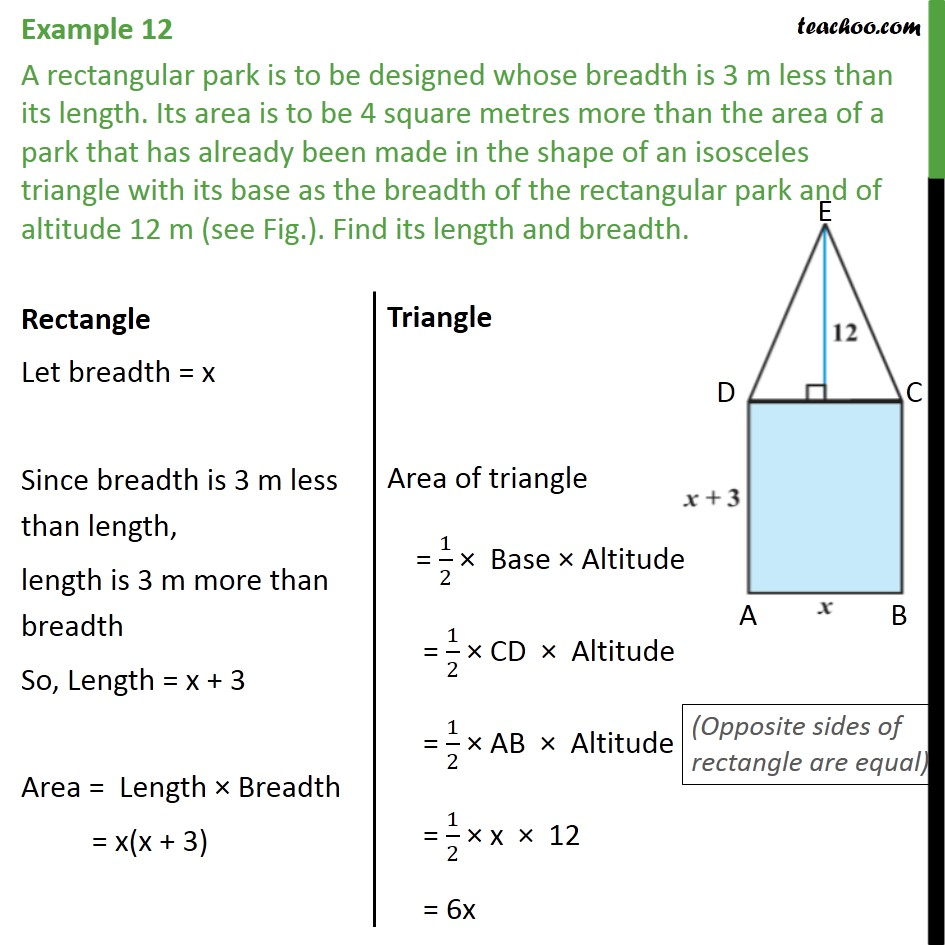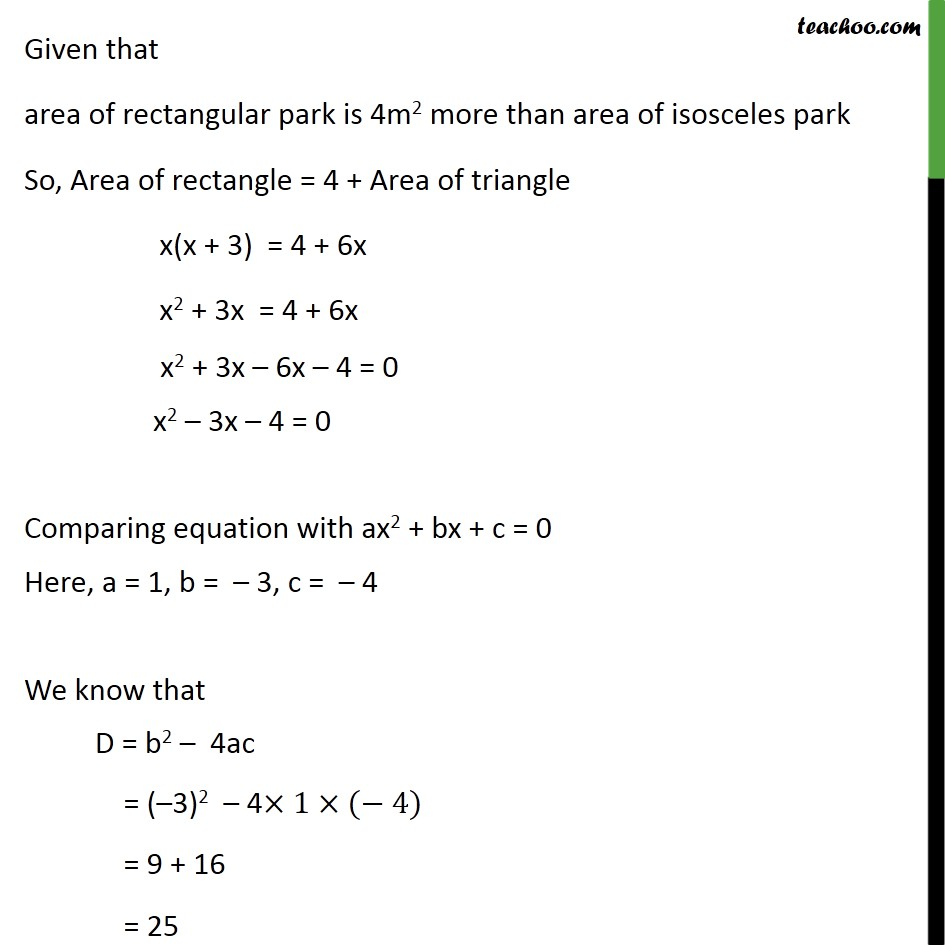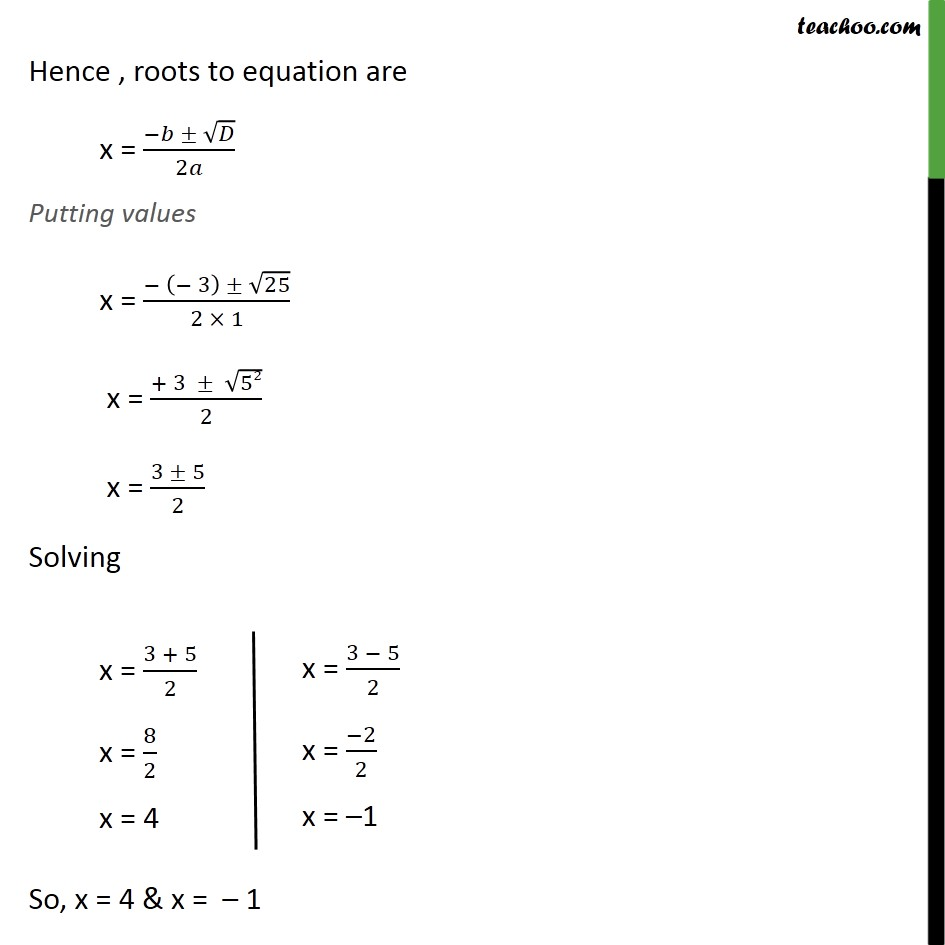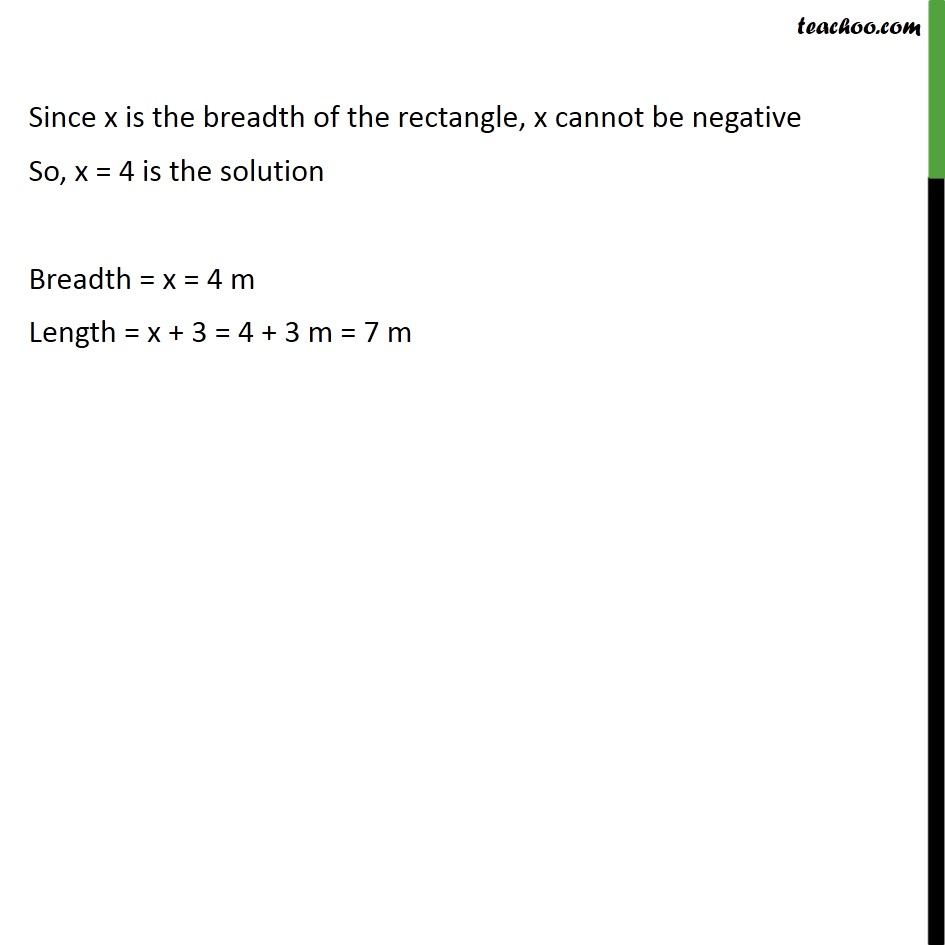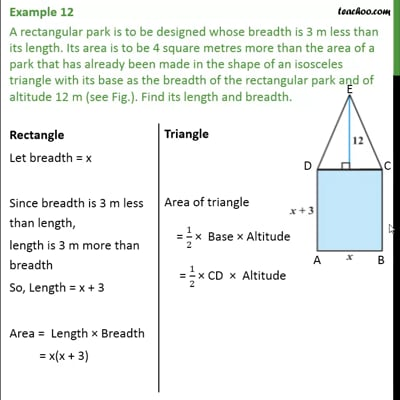This video is only available for Teachoo black users

Introducing your new favourite teacher - Teachoo Black, at only ₹83 per month

### Transcript

Example 12 A rectangular park is to be designed whose breadth is 3 m less than its length. Its area is to be 4 square metres more than the area of a park that has already been made in the shape of an isosceles triangle with its base as the breadth of the rectangular park and of altitude 12 m (see Fig.). Find its length and breadth. Given that area of rectangular park is 4m2 more than area of isosceles park So, Area of rectangle = 4 + Area of triangle x(x + 3) = 4 + 6x x2 + 3x = 4 + 6x x2 + 3x 6x 4 = 0 x2 3x 4 = 0 Comparing equation with ax2 + bx + c = 0 Here, a = 1, b = 3, c = 4 We know that D = b2 4ac = ( 3)2 4 1 ( 4) = 9 + 16 = 25 Hence , roots to equation are x = ( )/2 Putting values x = ( ( 3) 25)/(2 1) x = (+ 3 52)/2 x = (3 5)/2 Solving So, x = 4 & x = 1 Since x is the breadth of the rectangle, x cannot be negative So, x = 4 is the solution Breadth = x = 4 m Length = x + 3 = 4 + 3 m = 7 m